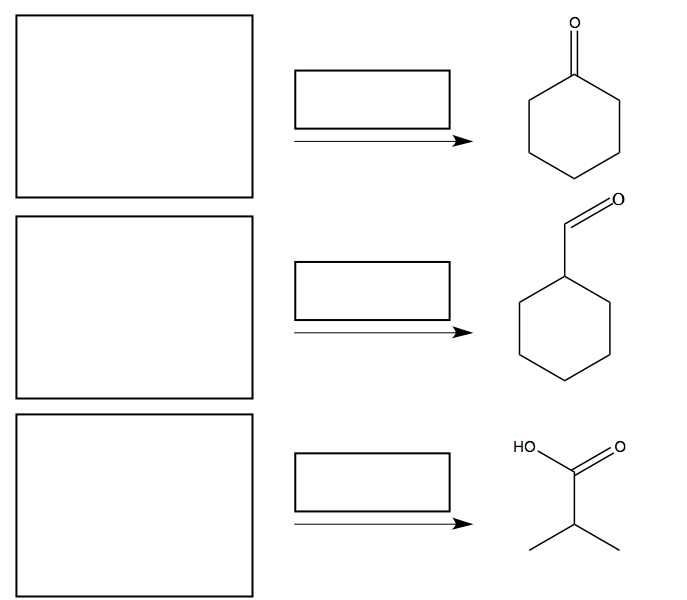# Problem: Draw the structure of the alcohols, which are the direct precursors of the following carbonyl derivatives. Which reagent would you use to achieve these transformations?

###### FREE Expert Solution
82% (342 ratings)
###### Problem Details

Draw the structure of the alcohols, which are the direct precursors of the following carbonyl derivatives. Which reagent would you use to achieve these transformations?Frequently Asked Questions

What scientific concept do you need to know in order to solve this problem?

Our tutors have indicated that to solve this problem you will need to apply the Oxidizing Agent concept. You can view video lessons to learn Oxidizing Agent. Or if you need more Oxidizing Agent practice, you can also practice Oxidizing Agent practice problems.

What is the difficulty of this problem?

Our tutors rated the difficulty ofDraw the structure of the alcohols, which are the direct pre...as medium difficulty.

How long does this problem take to solve?

Our expert Organic tutor, Chris took 4 minutes and 8 seconds to solve this problem. You can follow their steps in the video explanation above.